Related Articles

# Types of Autocorrelation

• Last Updated : 17 May, 2021

#### Autocorrelation:

As we discussed in this article, Autocorrelation is defined as the measure of the degree of similarity between a given time series and the lagged version of that time series over successive time periods. Autocorrelation measures the degree of similarity between a time series and the lagged version of that time series at different intervals.

#### Autocorrelation Function:

Suppose we have a time series {Xt} which has the following mean:and the autocovariance functionsat t=0,and the autocorrelation function is defined as:The value of autocorrelation varies from -1 for perfectly negative autocorrelation and 1 for perfectly positive autocorrelation. The value closer to 0 is referred to as no autocorrelation.

#### Positive Autocorrelation:

Positive autocorrelation occurs when an error of a given sign between two values of time series lagged by k followed by an error of the same sign.Below is the graph of the dataset that represents positive autocorrelation at lag=1: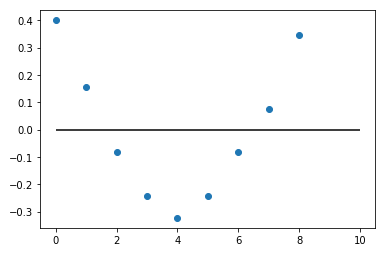#### Negative Autocorrelation:

Negative autocorrelation occurs when an error of a given sign between two values of time series lagged by k followed by an error of the different sign.Below is the graph of time series that represents negative autocorrelation at lag=1: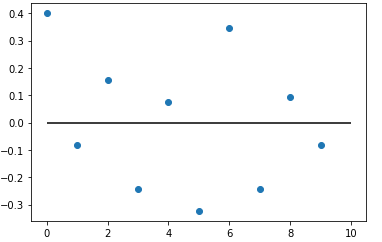#### Strong Autocorrelation

We can conclude that the data have strong autocorrelation if the autocorrelation plot has similar to the following plots: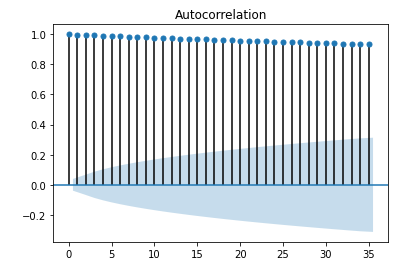The autocorrelation plot starts with a very high autocorrelation at lag 1 but slowly declines until it becomes negative and starts showing an increasing negative autocorrelation. This type of pattern indicates a strong autocorrelation, which can be helpful in predicting future trends

The next step would be to estimate the parameters for the autoregressive model:The randomness assumption for least-squares fitting applies to the residuals of the model. That is, even though the original data exhibit non-randomness, the residuals after fitting Yi against Yi-1 should result in random residuals.

#### Weak Autocorrelation

We can conclude that the data have weak autocorrelation if the autocorrelation plot has similar to the following plot at lag = 1: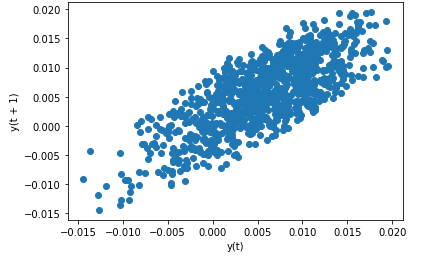Lag plot at Lag =1

The above plot shows that there is some autocorrelation at lag=1 because if there is no autocorrelation the plot will be similar to this plot on random values with lag=1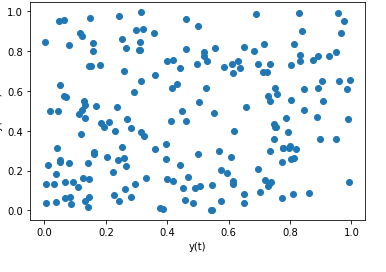The conclusion can be drawn from the above plot

• An underlying autoregressive model with moderate positive/negative autocorrelation.
• There were very few outliers.

The above weak autocorrelation plot have some autoregressive model that can be represented in such a format Yi =0, we can obtain the residual of estimators.It is easy to perform estimation on the lag plot because of the Yi+1 and Yi as their axes.

#### Implementation

• In this implementation, we will be looking on how to generate correlation plots and lag plots. We will use the flicker dataset and some randomly generated samples for this purpose.

## python3

 # Necessary importsimport numpy as npfrom numpy.random import random_sampleimport pandas as pdimport matplotlib.pyplot as pltimport statsmodels.api as smfrom statsmodels.graphics.tsaplots import plot_acf # Generate Autocorrelation plot at different lags# with a given level of significance.weak_Corr_df = pd.read_csv('flicker.csv', sep ='\n', header=None)plot_acf(weak_Corr_df, alpha = 0.05) # Generate Lag plots for a particular lag valuepd.plotting.lag_plot(weak_Corr_df, lag = 1) # Generate 200 random numbers and plot lag plot and autocorrelation plot for thatrandom_Series = pd.Series(random_sample(200))pd.plotting.lag_plot(random_Series, lag = 1)plot_acf(random_Series, alpha = 0.05)
• For Flicker dataset, the plots are as follows: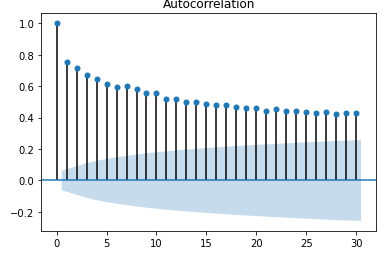Autocorrelation Flicker dataLag plot for flicker data

• For Random Normal datasets, the plots are as follows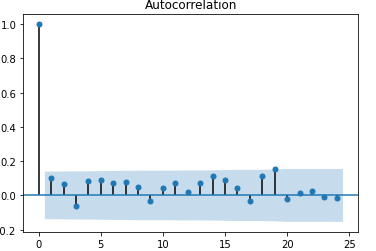Autocorrelation plotLag plot for random data at lag=1

Attention reader! Don’t stop learning now. Get hold of all the important Machine Learning Concepts with the Machine Learning Foundation Course at a student-friendly price and become industry ready.

My Personal Notes arrow_drop_up